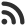Feed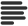Articles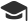Tutorials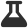CodingLab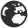CommunityLeaderboardvishal_saxena published an article 2 months ago

# Find maximum xor of k elements in an array

Given an array arr[] of N integers and an integer K. The task is to find the maximum xor subset of size K of the given array.

Lets take a look at an example.

Input

``arr[] = {2, 5, 4, 1, 3, 7, 6, 8}, K = 3``

Output

``15``

Explanation

We can get 15 by selecting 4, 5, 6, 8

## Naive approach

Iterate over all subsets of size K of the array and find maximum xor among them.

## Implementation

``````// C++ implementation

#include <bits/stdc++.h>
using namespace std;

// Function to return the maximum xor for a
// subset of size k from the given array
int Max_Xor(int arr[], int n, int k)
{

// Initialize result
int maxXor = INT_MIN;

// Traverse all subsets of the array
for (int i = 0; i < (1 << n); i++) {

// __builtin_popcount() returns the number
// of sets bits in an integer
if (__builtin_popcount(i) == k) {

// Initialize current xor as 0
int cur_xor = 0;
for (int j = 0; j < n; j++) {

// If jth bit is set in i then include
// jth element in the current xor
if (i & (1 << j))
cur_xor = cur_xor ^ arr[j];
}

// Update maximum xor so far
maxXor = max(maxXor, cur_xor);
}
}
return maxXor;
}

// Main Function
int main()
{
int arr[] = { 2, 5, 4, 1, 3, 7, 6, 8 };
int n = sizeof(arr) / sizeof(int);
int k = 3;

cout << Max_Xor(arr, n, k);

return 0;
}
``````

``15``

## Efficient approach

The problem can be solved using dynamic programming. Create a dp table dp[i][j][mask] which stores the maximum xor possible at the ith index (with or without including it) and j denotes the number of remaining elements we can include in our subset of K elements. Mask is the xor of all the elements selected till the ith index. This approach will only work for smaller arrays due to space requirements for the dp array.

## Implementation

``````// C++ implementation

#include <bits/stdc++.h>
using namespace std;

#define MAX 10000
#define MAX_ELEMENT 50

int dp[MAX_ELEMENT][MAX_ELEMENT][MAX];

// Function to return the maximum xor for a
// subset of size k from the given array
int Max_Xor(int arr[], int i, int j, int mask, int n)
{
if (i >= n) {

// If the subset is complete then return
// the xor value of the selected elements
if (j == 0)
else
return 0;
}

// Return if already calculated for some
// mask and j at the i'th index

int ans = 0;

// If we can still include elements in our subset
// include the i'th element
if (j > 0)
ans = Max_Xor(arr, i + 1, j - 1, mask ^ arr[i], n);

// Exclude the i'th element
// ans store the max of both operations
ans = max(ans, Max_Xor(arr, i + 1, j, mask, n));

}

// Main Function
int main()
{
int arr[] = { 2, 5, 4, 1, 3, 7, 6, 8 };
int n = sizeof(arr) / sizeof(int);
int k = 3;

memset(dp, -1, sizeof(dp));

cout << Max_Xor(arr, 0, k, 0, n);

return 0;
}
``````

``15``

 arrow_upward UpvoteComment arrow_downward downvote

Events

Nov. 28, 2018, 5:30 p.m.

Python from zero to hero

place Delhi

Aug. 13, 2018, 5:30 p.m.

Python from zero to hero

place Bangalore ( HackersFriend office BTM Layout)